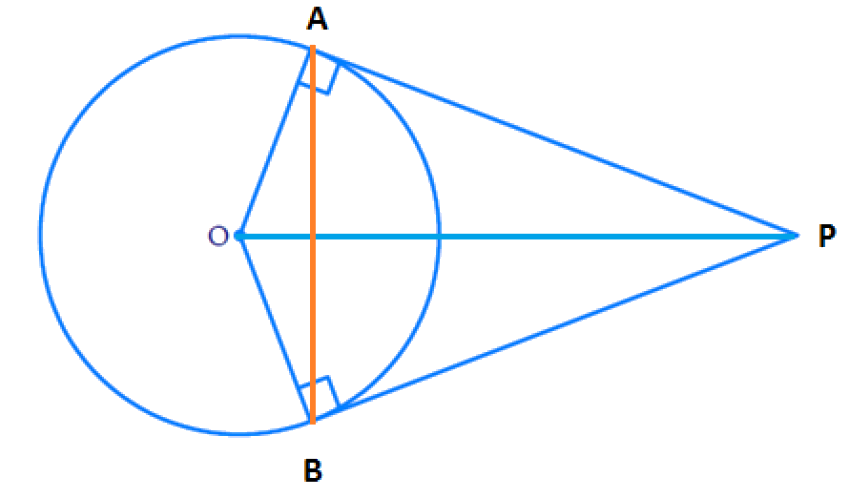# Ex.10.2 Q10 Circles Solution - NCERT Maths Class 10

Go back to  'Ex.10.2'

## Question

Prove that the angle between the two tangents drawn from an external point to a circle is supplementary to the angle subtended by the line-segment joining the points of contact at the center.

Video Solution
Circles
Ex 10.2 | Question 10

## Text Solution

What is Known?

• Let us consider a circle centered at point $${O.}$$
• Let $${P}$$ be an external point from which two tangents $${PA}$$ and $${PB}$$ are drawn to the circle which are touching the circle at point $${A}$$ and $${B}$$ respectively
• $${AB}$$ is the line segment, joining point of contacts $${A}$$ and $${B}$$ together such that it subtends $$\angle {AOB}$$ at center $${O}$$ of the circle.

To prove:

The angle between the two tangents drawn from an external point to a circle is supplementary to the angle subtended by the line segment joining the point of contact at the center.

i.e. $$\angle {APB}$$ is supplementary to $$\angle {AOB}$$

Reasoning:According to Theorem 10.1: The tangent at any point of a circle is perpendicular to the radius through the point of contact.

\begin{align}\therefore \angle {OAP} = \angle {OBP} = 90^{ \circ } \ldots \end{align}  Equation (i)

Steps :

In a quadrilateral, sum of 4 angles is $${360^ \circ }$$

$$\therefore$$ In $${OAPB}$$,

\begin{align}\begin{bmatrix}\angle {OAP} + \angle {APB} + \\\angle {PBO} + \angle {BOA} \end{bmatrix}= 360 ^ { \circ }\end{align}

Using Equation (i), we can write the above equation as

\begin{align} \left[ \begin{array} & {{90}^{{}^\circ }}+\angle APB+ \\ {{90}^{{}^\circ }}+\angle BOA \\ \end{array} \right]&\!\!=\!{{360}^{^\circ }} \\ \angle APB+\angle BOA&\!\!=\!{{360}^{{}^\circ }}\!\!-\!\!{{180}^{{}^\circ }} \\\therefore \angle APB+\angle BOA&\!\!=\!\!{{180}^{{}^\circ }} \\ \end{align}

Where, $$\angle {APB}=$$ Angle between the two tangents $${PA}$$ and $${PB}$$ from external point $${P}$$.

$$\angle {BOA}=$$ Angle subtended by the line segment joining the point of contact at the center.

Hence Proved.

Learn from the best math teachers and top your exams

• Live one on one classroom and doubt clearing
• Practice worksheets in and after class for conceptual clarity
• Personalized curriculum to keep up with school